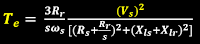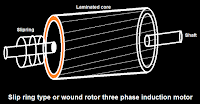# speed control methods of three phase induction motor?

249 views
speed control methods of three phase induction motor?

Speed control of three phase induction motor

•There are two types of AC motor one is synchronous motor and second is asynchronous motor (induction motor).

•Three phase induction motor is a self starting motor.

•Motor have two main parts one is stator and second is rotor.

•Speed of three phase induction motor can be control from stator side and rotor side.

•The main method for controlling speed of induction motor are one is by changing number of poles second is by stator voltage control third is by V/F control and fourth is by rotor resistance control.

By changing number of poles :

•This method is use for squirrel cage rotor motor only.•The number of stator poles can be change by three methods one is multiple stator winding and second is method of consequent poles and third is pole amplitude modulation.

•We have,"    N_s =  120f/P

•Where, N_s  is the synchronous speed , f is the frequency , P is the number of poles.

•So by changing the number of poles synchronous speed changes.

By stator voltage control :

•We have,•Torque is directly proportional to stator voltage.

•This method is very easy and cheap but still use rarely.

•Because by decreasing the voltage torque will reduce.

•But this will increase current in motor which will heat the motor which is not good it causes losses.

•Also the torque is proportional to square of voltage so large voltage change is required for changing torque.

By V/F control :

•We have,  N_s=  120f/P

•Where, N_s  is the synchronous speed , f is the frequency , P is the number of poles.

•Also, V=4.44φfTK  ( which means φ α V/f ).

•Where, V is the voltage , φ is the flux , f is the frequency , T is number of turns per phase , K is the winding constant.

•If we increase the frequency (f) flux (φ) will decrease because they are inversely proportional and if we increase the voltage (V ) flux (φ) will increase because they are directly proportional.

•In this method we have to keep V/f ratio constant.

By Rotor resistance control :

•We have, Torque α slip/(rotor resistance)

•This method is use for wound rotor motor only.•By adding external variable resistance to rotor via slip ring we can control the speed of motor.

•It can also use as starter.

•It is simple method.

•But there is resistance means heat means losses means less efficiency.

## Related Questions

140 views

Description : The speed of a three-phase induction motor is controlled by variable voltage variable frequency control (i.e. keeping V/f constant). As the frequency is reduced, the slip at maximum torque  (1) Decreases (2) Increases (3) Remains constant (4) None of the above

Last Answer : The speed of a three-phase induction motor is controlled by variable voltage variable frequency control (i.e. keeping V/f constant). As the frequency is reduced, the slip at maximum torque Increases

160 views

Description : What are the speed control methods of dc motor?

Last Answer : Field control of DC motor Armature control of DC motor

251 views

Description : The slip of 400 V, three phase, 4 -pole induction motor when rotating at 1440 rpm is

Last Answer : The slip of 400 V, three phase, 4 -pole induction motor when rotating at 1440 rpm is 4 percent

108 views

Description : How is a three phase cage induction motor self starting?

Last Answer : Any type of induction motor is self starting. Because the reason for torqe production require two flux displaced by some angle theta.If theta is constant with time then torqe will be constant;if ... torqe also pulsating. In induction motor this angle theta is not pulsating.so it is self starting